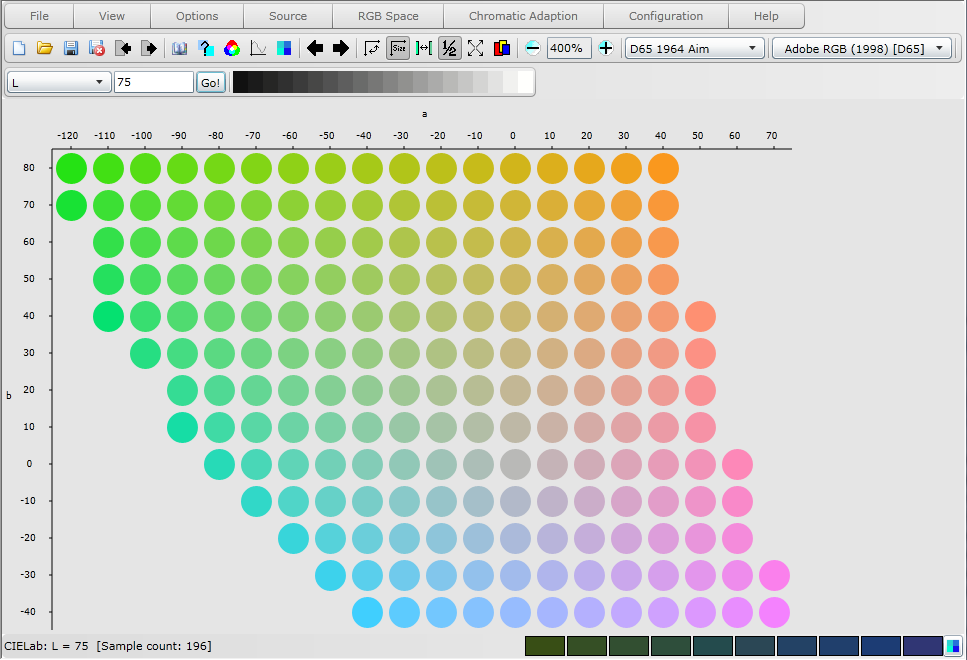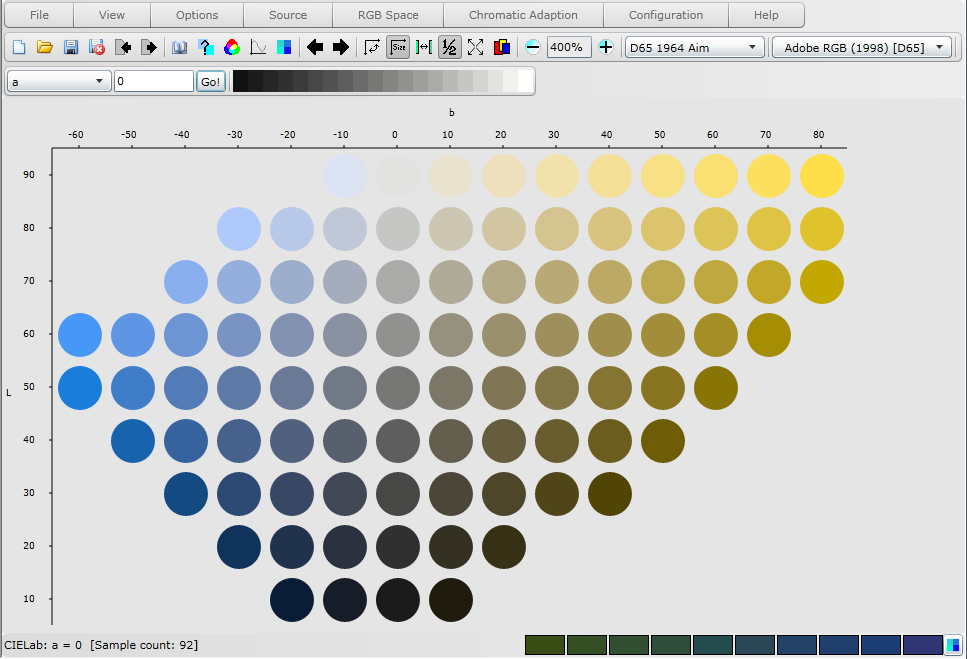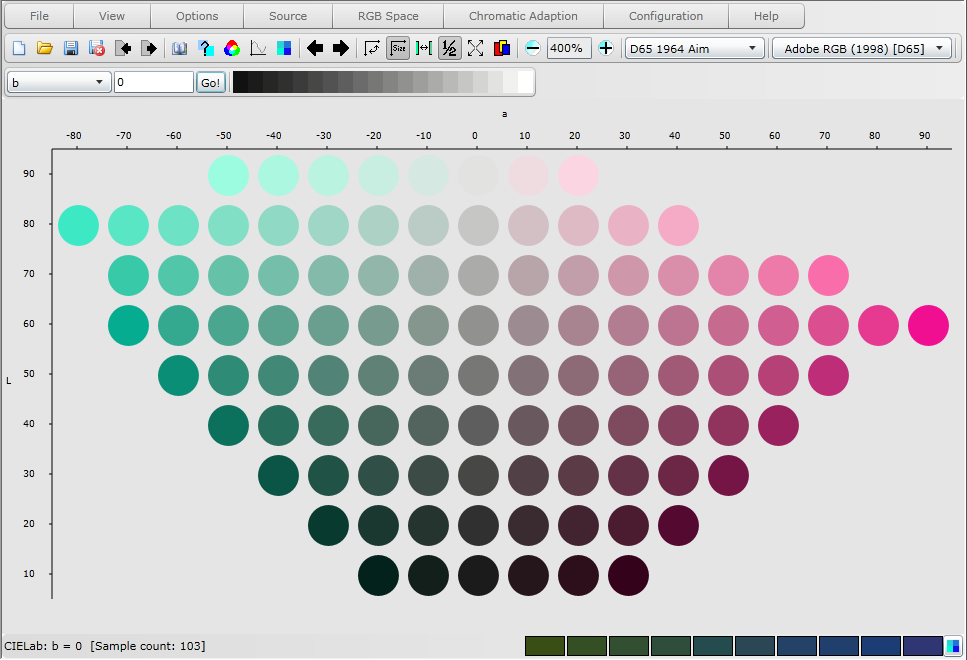Virtual Colour Systems Ltd. Virtual Atlas Home Help Menu
 CIELab The CIELab system is derived from Adams's (1942) system, the colour difference formula being a cube root version of the Adams-Nickerson formula. The colour difference formula developed by Adams is usually referred to as the Adams-Nickerson colour difference formula due to the work of Nickerson (1947,1950) which led to the successful introduction of the formula in industrial applications. The rectangular coordinates L*,a*,b* of this system are calculated from the CIE X,Y,Z tristimulus values by equations. Lightness forms the central axis of the system with the a and b axes perpendicular to the lightness axis and each other. Planes of constant lightness form ab chromaticity planes.The a axis approximates to the red-green axis and varies in extent based on Lightness.The b axis approximates to the blue-yellow axis and varies in extent based on Lightness.Copyright 2011, Virtual Colour Systems Ltd. All Rights Reserved